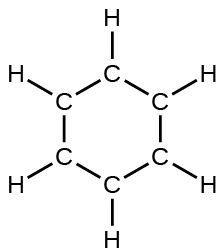# Problem: Write resonance forms that describe the distribution of electrons in each of these molecules or ions.benzene, C6H6:

###### FREE Expert Solution

We are being asked to identify the resonance forms that describe the distribution of electrons in benzene, C6H6.

First, we will have to draw the Lewis Structure of benzene.

Step 1: Determine the central atom in this molecule.

H is less electronegative than C but H can only make one bond

• C would also bond with either C or H forming a total of 4 bonds each C atom

• C would be the central atom

Step 2: Calculate the total number of valence electrons present.

C6H6:

Group              Valence Electrons

C         4A                    4 valence e- x 6
H         1A
1 valence e- x 1
Total =
30 valence e-

Step 3: Draw the Lewis Structure for the molecule

81% (44 ratings)###### Problem Details

Write resonance forms that describe the distribution of electrons in each of these molecules or ions.

benzene, C6H6